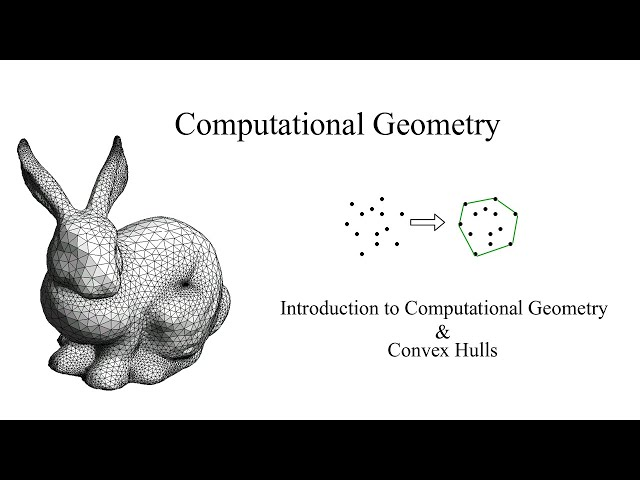# What is Geometric algorithms

##### Exploring Geometric Algorithms

Geometry is a branch of mathematics that studies the properties and relationships of points, lines, shapes, and surfaces in two and three-dimensional space. Geometric algorithms are computer algorithms designed to solve various problems in geometry such as finding the intersection of two shapes, calculating the distance between two points, and measuring the area of a shape. In this article, we will explore the different types and applications of geometric algorithms.

##### Types of Geometric Algorithms

Geometric algorithms can be classified into several categories based on their scope and application. The following are some of the most common types of geometric algorithms:

• Point Location Algorithms: These algorithms are used to determine the position of a given point relative to a set of geometric shapes such as lines or polygons. They are commonly used in computer graphics and computer-aided design (CAD) applications.
• Convex Hull Algorithms: These algorithms are used to find the convex hull of a set of points or a shape. The convex hull is the smallest convex polygon that encloses all the points in the set. This type of algorithm is used in spatial data analysis and computational geometry.
• Triangulation Algorithms: These algorithms are used to partition a shape or a set of points into a triangle mesh. Triangulation algorithms are commonly used in computer graphics, computer vision, and finite element analysis.
• Intersection Detection Algorithms: These algorithms are used to determine if two shapes intersect and if so, where they intersect. Intersection detection algorithms are widely used in CAD, image processing, and robotics applications.
• Distance Calculations: These algorithms are used to calculate the distance between two shapes or points. Distance calculation algorithms are essential in GIS, remote sensing, and image processing applications.
##### Applications of Geometric Algorithms

Geometric algorithms are used in various applications such as computer graphics, robotics, computer-aided design (CAD), and computational geometry. The following are some of the most common applications of geometric algorithms:

• Computer Graphics: Geometric algorithms are used in computer graphics to create 3D models, render images, and simulate physics. They are also used to process and display 2D and 3D data in real-time.
• Robotics: Geometric algorithms play a critical role in robotic systems for navigation, perception, and motion planning. They are used to map environments, detect obstacles, and plan optimal paths for robots.
• Computer-Aided Design: Geometric algorithms are used in CAD software to create, edit, and manipulate 2D and 3D geometries. They are also used to perform geometric analysis and optimization tasks.
• Computational Geometry: Geometric algorithms are used in computational geometry to solve geometric problems such as proximity queries, convex hulls, and Voronoi diagrams. They are also used in mesh generation and optimization.
• GIS and Remote Sensing: Geometric algorithms are used in GIS and remote sensing to process and analyze spatial data. They are used to calculate distances, areas, and volumes, as well as to detect changes in land cover and land use over time.
##### Challenges in Geometric Algorithms

Geometric algorithms pose several challenges that make them difficult to design and implement. The following are some of the most common challenges in geometric algorithms:

• Numerical Instability: Geometric algorithms often involve floating-point arithmetic operations that can be sensitive to small changes in input data. This can lead to numerical instability and numerical errors.
• Robustness: Geometric algorithms need to be robust and handle various input data types, shapes, and sizes. They also need to be able to handle degenerate cases such as collinear points and coincident edges.
• Complexity: Geometric algorithms can have high computational complexity due to the large input sizes and the need to perform geometric operations repeatedly.
• Data Structures: Geometric algorithms often require efficient data structures such as quad-trees, kd-trees, and Voronoi diagrams to store and manipulate geometric data.
• Parallelization: Geometric algorithms can benefit from parallel processing to reduce the computation time and increase the scalability of the algorithm.
##### Conclusion

Geometric algorithms are an essential component of computer science and mathematics. They have a wide range of applications in various fields such as computer graphics, robotics, and GIS. Developing efficient and robust geometric algorithms that can handle complex input data types and sizes is a challenging task that requires a deep understanding of computational geometry. As the field of computer science and mathematics continues to evolve, geometric algorithms will continue to play a critical role in solving various problems and advancing technology.